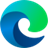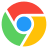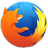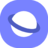# calc() CSS Function

### Description

The CSS `calc()` function is a powerful tool that allows you to perform mathematical calculations on CSS property values. It can be used with any numeric CSS property, including `width`, `height`, `margin`, `padding`, `font-size`, and more.

The `calc()` function takes a single expression as its argument, which can be any combination of numbers, CSS variables, and mathematical operators. The result of the expression is then used as the value of the CSS property.

Here are some examples of how to use the `calc()` function:

``````/* Set the width of an element to 50% of its parent element's width */
width: calc(50%);

/* Set the margin of an element to 10 pixels on all sides */
margin: calc(10px);

/* Set the font-size of an element to 16 pixels plus the value of the `\$font-size` variable */
font-size: calc(16px + \$font-size);

/* Set the width of an element to the width of the viewport minus 200 pixels */
width: calc(100vw - 200px);
``````

The `calc()` function is a very versatile tool that can be used to create all sorts of dynamic and responsive CSS layouts. It is also very well supported by modern browsers.

Here are some of the benefits of using the `calc()` function:

• It allows you to create more dynamic and responsive CSS layouts.
• It can be used to simplify your CSS code and make it more maintainable.
• It is well supported by modern browsers.

If you are not already using the `calc()` function in your CSS, I encourage you to give it a try. It is a powerful tool that can help you to create more efficient and maintainable CSS code.

### Syntax

```<calc()> = calc( <calc-sum> )
<calc-sum> = <calc-product> [ [ '+' | '-' ] <calc-product> ] *
<calc-product> = <calc-value> [ '*' <calc-value> | '/' <calc-number-value> ] *
<calc-value> = <number> | <dimension> | <percentage> | ( <calc-sum> )
<calc-number-sum> = <calc-number-product> [ [ '+' | '-' ] <calc-number-product> ] *
<calc-number-product> = <calc-number-value> [ '*' <calc-number-value> | '/' <calc-number-value> ] *
<calc-number-value> = <number> | ( <calc-number-sum> )```

### Values

• +addition (width: calc (20px + 20px));
• -subtraction (padding: calc (10% - 10px););
• *multiplication (height: calc (20% * 2);)
• /division. It is forbidden to divide by zero (width: calc (100% / 3);)

### Example

``<div class="boxcalc">Example using the calc() CSS function.</div>``
``````.boxcalc {
width: calc(100% - 100px);
border: 2px solid red;
background-color: #ccc;
The following table will show you the current browser support for the CSS `calc()` function.
 Desktop12 26 16 15 7
 Tablets / Mobile28 16 14 7 1.5 37